Womxn in Big Data South Africa: Female-Headed Households in South Africa Challenge Tutorial
23 Jun 2022, 14:05

This is a step-by-step approach on how to approach the Womxn in Big Data South Africa: Female-Headed Households in South Africa Challenge. The article will take you through the basic procedure on how best to approach this task, show you some basic techniques you can use and finally show you how to create and upload your submission to Zindi for scoring.

Use this colab notebook to follow through.

The complete description of the challenge can be found here.

Objective of the challenge

The objective of this challenge is to build a predictive model that accurately estimates the % of households per ward that are female-headed and living below a particular income threshold by using data points that can be collected through other means without an intensive household survey like the census.

Type of Problem

From the description above, we know that we are building a Regression Model.

Regression is a subset of supervised learning that aims to predict a continuous or numerical value.

Our goal is to build a predictive model that estimates the % of households per ward female-headed and living below a particular income threshold by using data points that can be collected through other means without an intensive household survey like a census.

Data Understanding

This involves collecting data, assessing its quality and transforming variables into their correct formats.

1. Set up your work environment and Import Data

`# Install Catboost model`
`!pip install catboost -q`

`# Import libraries`
`import pandas as pd`
`import numpy as np`
`import re`
`import matplotlib.pyplot as plt`
`import seaborn as sns`
`import requests`
`import plotly.express as px`
`from sklearn.metrics import mean_squared_error`
`from sklearn.model_selection import train_test_split, KFold`
`from catboost import CatBoostRegressor`
`from IPython.display import display`
`pd.set_option('display.max_columns', None)`
`pd.set_option('display.max_rows', None)`
`pd.set_option('max_colwidth', None)`
`%matplotlib inline`
`import warnings`
`warnings.filterwarnings('ignore')`

Next, we check the data’s quality and completeness, exploration of variables and their relationship and get a brief description of the data. Things you need to look out for in the different variables from our dataset are:

1. Variable types are in the correct format.
2. The variables - think about the features to use
3. If you need more detailed statistics - use the describe() function
4. Rename the variables if they look wonky

For this, the variable_definitions.csv describes the different variables in the data.

`# Load files`
`train = pd.read_csv('Train.csv')`
`test = pd.read_csv('Test.csv')`
`sample_submission = pd.read_csv('SampleSubmission.csv')`
`variable_definations = pd.read_csv('variable_descriptions.csv')`

Exploratory Data Analysis

For data exploration and understanding, The Pandas library built on top of Python thrives in data manipulation and analysis by providing data structures (Series: one-dimensional object and DataFrame: Two-dimensional object) and offers operations for manipulating numerical tables.

Check for missing values:

`# Check for missing values`
`train.isnull().sum().any(), test.isnull().sum().any()`

Check for duplicated data:

`# Check for duplicates`
`train.duplicated().any(), test.duplicated().any()`

Creating visualizations from our dataset libraries like Seaborn, or Matplotlib can be helpful.

Plotting the distribution of the target:

`# target distribution`
`plt.figure(figsize = (11, 5))`
`sns.histplot(train.target)`
`plt.title('Target Distribution')`
`plt.show()`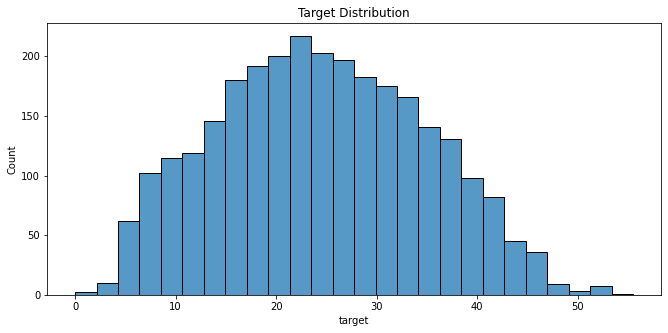From the target distribution histogram above we can see that the distribution follows a normal curve, in that it is neither skewed to the left nor the right.

This is good news for a regression task. If the distribution was skewed, preprocessing and post-processing of the target would have been necessary.

Check the distribution of female-headed households across South Africa:

`# get SouthAfrica  municipal boundaries`
`res = requests.get( "https://raw.githubusercontent.com/deldersveld/topojson/master/countries/south-africa/south-africa-provinces.json")`
`# scatter the cities and add layer that shows municiple boundary`
`px.scatter_mapbox(train, lat="lat", lon="lon", hover_name="target").update_layout(mapbox={"style": "carto-positron","zoom": 4.5,"layers": [{`
`               "source": res.json(),`
`               "type": "line",`
`               "color": "green",`
`               "line": {"width": 1},}],})`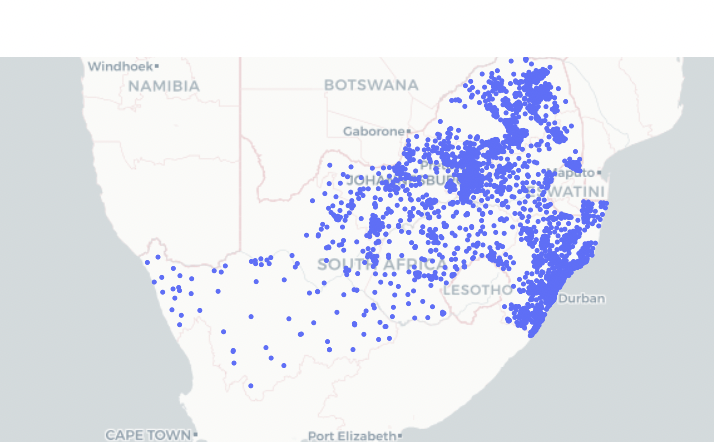• From the map above we can see that there is a high concentration of female-headed households in the North East and South East of South Africa
• The West of South Africa doesn't have many female-headed households as compared to the East

Check whether the target variable has any outliers:

`# Check for outliers in the target variable`
`plt.figure(figsize = (11, 5))`
`sns.boxplot(train.target)`
`plt.title('Boxplot showing outliers - target variable')`
`plt.show()`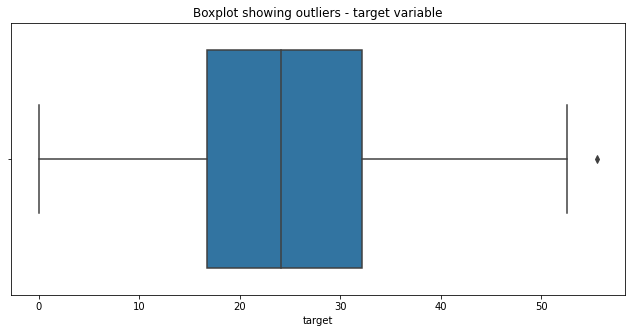The target variable has some outliers that are beyond the 50 mark.

Outliers can be handled via

• Dropping them
• Cap outliers - set a maximum
• Assign a new value to the outliers
• Transform the target variable

Correlation check

Correlation Analysis is super useful when you want to understand the relationship among variables (columns). It's important because when we know a score on one measure, we can make a more accurate prediction of another highly related measure. The stronger the relationship between/among variables the more accurate the prediction.

The next step is to understand the correlation in our dataset:

`# Correlation of some of the dwelling variables`
`plt.figure(figsize= (13, 7))`
`sns.heatmap(train[train.columns[:15]].corr(), annot = True, cmap='rainbow',linewidths=0.1,vmax=1.0, linecolor='white', square=False)`
`plt.title('Heatmap showing correlation between dwellings variables', color = 'm', pad = 15, fontdict={'size': 15})`
`plt.show()`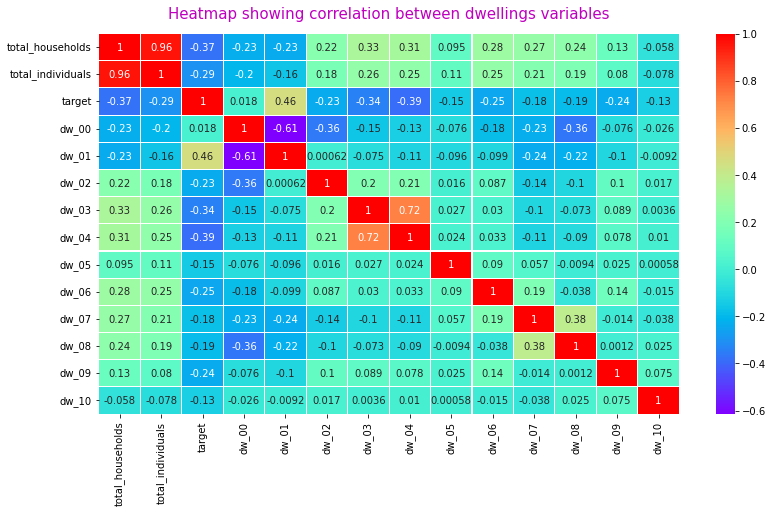There is a strong positive correlation of 0.72 between dwelling dw_03 and dw_04 - Why is this? More investigation is needed

• dw_03 - Percentage of dwellings of type: Cluster house in the complex
• dw_04 - Percentage of dwellings of type: Townhouse (semi-detached house in a complex)

There is also a mildly strong negative correlation of -0.61 between dwelling dw_01 and dw_00

• dw_00 - Percentage of dwellings of type: House or brick/concrete block structure on a separate stand or yard or on a farm

Modeling

For this challenge, we will use the Catboost algorithm -it is an open-source gradient boosting algorithm developed by the Yandex team in 2017. It is a machine learning algorithm that allows users to quickly handle categorical features for a large data set and this differentiates it from XGBoost & LightGBM.

CatBoost algorithm is based on gradient boosting decision trees. Gradient boosting is a Machine learning technique used in classification and regression tasks. Boosting is a method of converting weak learners into strong learners - what happens is the technique combines models to produce one optimal predictive model.

Catboost can be used to solve regression, classification and ranking problems. Since this is a regression problem, we will use the CatBoostRegressor Algorithm. Here we are dropping the columns with no correlation with the target variable

Select Independent and dependant variables to be used in training:

`# Select X and y features`
`X = train.drop(['ward', 'target'], axis = 1)`
`y = train.target`
`test_df = test.drop('ward', axis = 1)`

Split the data into five folds using KFold to avoid overfitting:

`# KFold Validation`
`folds = KFold(n_splits = 5, shuffle=True, random_state = 42)`

Train the model per fold and make predictions on the test data:

` model.fit(X_train, y_train,`
`           eval_set=[(X_test, y_test)],`
`           early_stopping_rounds=10,`
`           verbose = 100,`
`           use_best_model = True)`

# Make predictions

` preds = model.predict(test_df)`
` y_pred = model.predict(X_test)`

After training, we get an average RMSE of 3.260. The chart below shows the most important features that contributed to the RMSE above.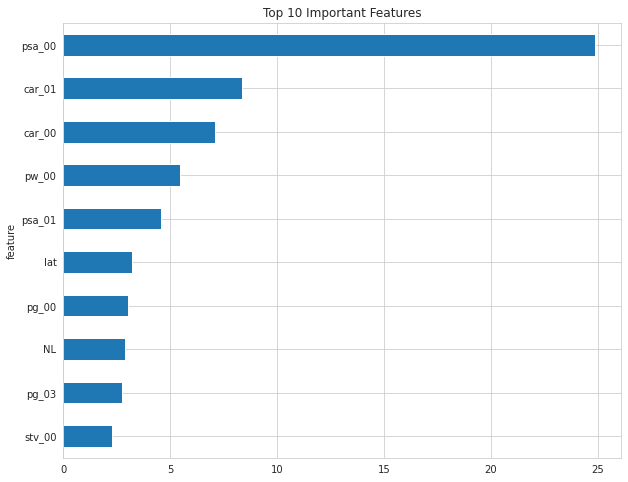Creating a submission file

When creating your submission - always look at the sample submission file to check whether it matches the format needed. When submitting your final predictions, always ensure that it's in CSV format.

`# Creating a submission file`
`mean_preds = np.mean(predictions, axis = 1)`
`sub_df = pd.DataFrame({'ward': test.ward, 'target': preds})`
`sub_df.head()`

Conclusion

Congratulations! You have understood the basic concepts of CatBoost Algorithm and built a model with the algorithm! Here are awesome code solutions:

Starter notebook by Zindi

Womxn in Big Data South Africa: Female-Headed Households in South Africa notebook